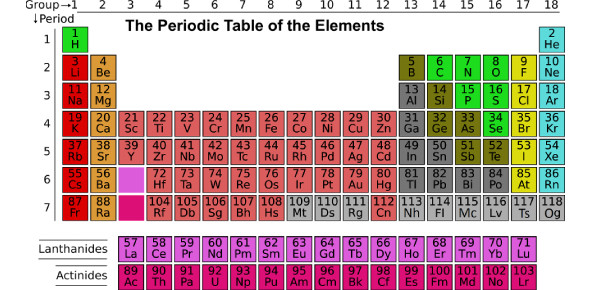Periodic Classification Of Elements - Paper I

10 Questions | Total Attempts: 4257SettingsTo identify the various trends in groups and periods To compare the traditional and modern methods of classification

• 1.
The electronic configuration of the atom of an element  X is 2 , 8, 4 .In the modern periodic table , the element X is placed in :
• A.

2 nd group

• B.

4 th group

• C.

14th group

• D.

8th group

• 2.
Which of the following element would lose an electron easily ?
• A.

Mg

• B.

Na

• C.

K

• D.

Ca

• 3.
The elements A, B, C, D and E have atomic numbers 9, 11 , 17,12, and 13 respectively . The pair of elements which belongs to the same group of the periodic table is :
• A.

A & B

• B.

B & D

• C.

A & C

• D.

D & E

• 4.
Which one of the following does not increase while moving down the group of the periodic table ?
• A.

• B.

Metallic character

• C.

Valence electrons

• D.

Shells in the atoms

• 5.
The Newlands Law of Octaves for the classification of elements was found to be applicable only up to the element :
• A.

Potassium

• B.

Calcium

• C.

Cobalt

• D.

Thorium

• 6.
An element  X  forms an oxide X2O3  . In which group of Modern periodic table is this element placed?
• A.

Group 2

• B.

Group 3

• C.

Group 5

• D.

Group 13

• 7.
The atomic numbers of the elements  Na,  Mg, K and Ca are 11, 12, 19, and 20 respectively. The element having the largest atomic radius is :
• A.

Mg

• B.

Na

• C.

K

• D.

Ca

• 8.
Where  would you locate the element with electronic configuration 2, 8 in the modern periodic table ?
• A.

Group 8

• B.

Group 18

• C.

Group 10

• D.

Group 16

• 9.
Which of the following is the valence shell for the elements of second period of the modern periodic table ?
• A.

M shell

• B.

K shell

• C.

L shell

• D.

N shell

• 10.
Which of the following statements is not a correct statement about the trends when going from left to right across the periods of the periodic table ?
• A.

The elements become less metallic in nature

• B.

The number of valence electrons increases

• C.

The atoms lose their electrons more easily

• D.

The oxides become more acidic

Related TopicsBack to top